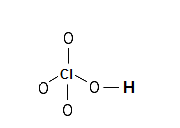Help with Lewis electron dot structures | Chlorine Perchlorate Cl2O4 | Chemistry Net

# Help with Lewis electron dot structures | Chlorine Perchlorate Cl2O4

Lewis Electron Dot Structures - Simple Procedure for writing Lewis Structures of chlorine perchlorate (Cl2O4)

# of chlorine perchlorate Cl2O4

A simple procedure for writing Lewis electron dot structures is given in a previous article entitled “Lewis Structures and the Octet Rule”. Several worked examples relevant to this procedure were given in previous posts please see the Sitemap - Table of Contents (Lewis Electron Dot Structures).

Another example  for writing Lewis structures following the above procedure is given below.

How can we construct the Lewis structure of Cl2O4?

Step 1: Connect the atoms with single bonds.Step 2: Calculate the # of electrons in π bonds (multiple bonds) using  formula (1) in the article entitled “Lewis Structures and the Octet Rule”.

Where n in this case is 6 since Cl2O4 consists of six atoms.

Where V = (6 + 7 + 6 * 2 + 6 + 7) = 38

Therefore, P = 6n + 2 – V = 6 * 6 + 2 – 38 = 0

So there are no π electrons in Cl2O4 and therefore the structure of Step 1 is the Lewis structure.

Electrons are placed around each atom so that the octet rule is obeyed. Formal charges are assigned and equalized using resonance.

Step 3 & 4: The Lewis structures for Cl2O4 are derived below:Relevant Posts - Relevant Videos

Lewis Structures|Octet Rule: A Simple Method to write Lewis Structures

Simple Method for writing Lewis Structures of Perchloric Acid HClO4

References

1. G.N. Lewis, J.A.C.S, 38, 762-785, (1916)
2. E. C. McGoran, J. Chem. Educ., 68, 19-23 (1991)
3. A.B.P. Lever, J. Chem. Educ., 49, 819-821, (1972)

Key Terms

resonance structures of chlorine perchlorate cl2o4, Lewis electron structures of chlorine perchlorate , chemical formula of chlorine perchlorate cl2o4, simple procedure for drawing Lewis structures of chlorine perchlorate,